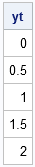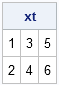When your data are in rows, but you need them in columns, use the matrix transpose function or operator. The same advice applies to data in columns that you want to be in rows.

For example, the vectors created by the DO function and the index creation operator are row vectors, but sometimes you might want to insert these sequential values into columns of a matrix. To convert a row of values into a column vector, you can use the T function, as follows:

```proc iml; y = do(0, 2, 0.5); /** linear sequence 0 to 2 by 0.5 **/ yt = T(y); print yt;```The transpose function also applies to two-dimensional matrices. The transpose of a two-dimensional matrix is obtained by flipping the matrix about its main diagonal. You can transpose square and nonsquare matrices, as shown in the following example:

```x = {1 2, 3 4, 5 6}; /** 3 x 2 matrix **/ xt = t(x); /** 2 x 3 matrix **/ print xt;```### The Transpose Operator: An Alternative Syntax

In mathematical and statistical books and articles, the transpose of a matrix X is often denoted by X' (read "X prime"). The SAS/IML language supports this notation through the transpose operator (`). This operator is typed by using the GRAVE ACCENT key. (The GRAVE ACCENT key is located in the upper left corner on US and UK QWERTY keyboards. Do not use the APOSTROPHE key, which is located next to the semicolon; In SAS software the APOSTROPHE key is used as a single quotation mark.) The operator transposes the matrix that it follows. For example, the following is an alternative statement which transposes the matrix x:

`xPrime = x`; /** alternative syntax for transpose **/`

When it occurs in a complicated formula, the transpose operator can be difficult to see. Therefore, some programmers prefer the clarity of using the more visible T function.

However, the transpose operator can sometimes result in better performance than using the T function because the SAS/IML language optimizes certain computations that involve matrix transposes. For example, the computation x` * x is more efficient than equivalent computation T(x) * x.

Programming Tip: The SAS/IML language optimizes certain computations that involve the transpose operator (`). Consequently, you should use the transpose operator instead of the T function when you are concerned about the speed of matrix computations.

The previous programming tip is one of more than 150 tips in my book Statistical Programming with SAS/IML Software.

Share